# 1 Models for Binary Responses

Consider first the Bernoulli distribution. For this, there are two possible outcomes, e..g. 0 and 1, or success and failure. For tosses of a fair coin, the probability of a Head is $$\frac{1}{2}$$. For tosses of an unbiased die, the probability of a six is $$\frac{1}{6}$$.

## 1.1 The Binomial and Poisson — R functions

The binomial distribution, with size $$n$$, is the distribution of the total number of 1’s (or ‘successes’) in $$n$$ independent Bernoulli trials with the same probability $$\pi$$. On the assumption that heads appear independently between coin tosses, with probability $$\pi$$ = 0.5 at each toss, the total number of heads in 10 tosses is binomial with size $$n$$ = 10 and $$\pi$$ = 0.5. The mean is $$n \pi = 5$$, while the variance is $$n \pi (1-\pi) = 2.5$$ .

There is no necessary reason why Bernoulli trials must be independent, or why the probability should be the same for all trials. As an example, if insects are exposed to a fumigant that is not inevitably fatal, it is unlikely that the probability of death will be the same for all insects. This has led to interest in other distributions, able to model a wider variety of types of data. In this vignette, the primary concern is with such alternatives.

Event processes lead to the Poisson distribution and its generalization. For an event process (e.g., radioactive decay events), the number of counts in any time interval will be Poisson if:

• Events occur independently – the occurrence of one event does not change the probability of a further event, and
• The rate $$\lambda$$ at which events occur is constant.

Thus, in radioactive decay, atoms appear to decay independently (and emit ionizing radiation), at a rate that is the same for all atoms. The mean is $$\lambda$$, which is also the variance. If the sample mean and the sample variance differ only by statistical error, data are to this extent consistent with a Poisson distribution.

Note that the Poisson distribution with rate $$\lambda$$ is the limiting distribution of the number of events (counting 1 or “success” as an event) for a binomial distribution as $$n$$ goes to infinity and $$\pi$$ goes to zero, with the binomial mean constant at $$n \pi = \lambda$$. It is, then, a limiting case of the binomial distribution.

Both for the binomial and for the Poisson, one parameter determines both the mean and the variance. This limits the data for which they can provide useful models, so that it becomes necessary to look for alternatives. The most commonly implemented alternative to the binomial is the betabinomial. There are a number of alternatives to the Poisson that have been widely implemented, with the negative binomial is the best known. See Rigby et al. (2019) for details of distributions that are implemented in the gamlss package.

For each distribution, there are four functions, with names whose first letter is one of d (density or, for discrete distributions, probability), p (cumulative probability), q (quantile), and r (generate a random sample). For an example, look ahead to the discussion of the binomial distribution in the next subsection. The following, using functions in the stats package that is by default available in R sessions, demonstrates the d/p/q/r nomenclature for the use of the binomial distribution to model the probability of 0, 1, 2, or 3 heads, in (size) 3 tosses, with probability (prob = 0.5) of a head. The functions dbinom() and pbinom() take number $$x$$ of heads as their first argument, and return (for dbinom()) a probability or (for pbinom()) a cumulative probability:

                                0     1     2     3
dbinom(x=0:3, size=3, prob=0.5) 0.125 0.375 0.375 0.125
pbinom(q=0:3, size=3, prob=0.5) 0.125 0.5   0.875 1    

Observe the different names, in the two cases, for the argument that gives the number of successes.

The inverse function to pbinom() is qbinom(). This takes as first argument a cumulative probability p, and returns the smallest value of $$x$$ such that pbinom(p) $$\leq$$ p.

                                                   0.125 0.5 0.875 1
qbinom(p=c(0.125,0.5,0.875,1.0), size=3, prob=0.5)     0   1     2 3

The result is unchanged if

• 0.125 is replaced by 0, or by any quantity between 0 and 0.125
• 0.5 is replaced by 0.126, or by any quantity $>$0.125 and $$\leq$$ 0.5
• 0.875 by 0.501, or …
• 1.0 by 0.876, or …

The inverse function, which determines quantiles, is defined to be 0 for any $$p \leq 0.125$$, stepping up to 1 for $$0.875 < p \leq 1.0$$. Figure 1.1 shows the (cumulative) distribution function, with the quantile function alongside: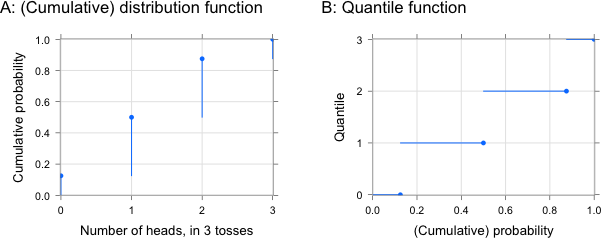Figure 1.1: Panel A shows the (cumulative) distribution function for the binomial. Panel B shows the inverse function, i.e., it plots the quantiles.

We would like to compare quantiles of a fitted distribution with quantiles of the data, as a mechanism for checking whether a model is a good fit to the data. For this, we prefer quantiles to be on a continuous dispersion. A complication, for the present data, and for other such discrete data, is that each of the horizontal lines in Figure 1.1B corresponds to a range of probabilities, thus:

x = Number of heads 0 1 2 3 Interval (0,0.125) (>0.125,0.5) (>0.5,0.875) (>0.875,1.0)

Functions such as qbinom() return the point that, moving from the vertical to the horizontal scale, is at the upper end of the relevant horizontal scale. Quantiles that incorporate a random point along the relevant horizontal line are preferable for use in model diagnostics, preferably repeating any check with several different sets of such randomized quantile residuals.’’ Depending on the number $$x$$ of diseased plants, choose $$u$$ to be a uniform random number in the corresponding interval shown above. If $$k=0$$, choose $$u$$ to be a uniform random number in the interval [0,0.125], and so on.

Then, if the model is correct, $$u$$ will be uniformly distributed on the interval $$0 <= u <=1$$. Given a set of values $$u_i$$, with $$i=1, \ldots m$$, these might be plotted against quantiles of the uniform distribution on the unit interval. It is, however, usual to transform to a standard normal distribution quantile scale. Thus 0.2 will translate to -0.84, 0.5 to 0, and 0.8 to 0.84.

round(qnorm(c(0.2,0.5,0.8)), 2)
 -0.84  0.00  0.84

Thus transformed, the quantiles can be plotted against quantiles of the normal distribution. Where a model has been fitted, the process is applied at each fitted value, generating “randomized quantile residuals.” The “randomized quantile residuals” are residuals, on a normal quantile scale, from the median of the fitted distribution.

# 2 More General Models for Binomial-like Counts

The glm() function in the stats package allows quasibinomial (and quasipoisson) families. This is not a formally defined distribution. Instead, they fit just as for the binomial or poisson, but estimate a dispersion from the fitted model that allows the variance to be larger (or, possibly, smaller) than the respective binomial or poisson variance.

The gamlss package implements, in each case, several alternatives. Parameters follow naming conventions that are different from those used for the stats package’s binomial family functions. The location parameter prob becomes mu for gamlss functions, while size becomes bd (= ‘bound’). The gamlss.dist package has an accompanying pdf “The gamlss.family distributions” that describes the distributions that the package makes available.

Bernoulli trials may not be independent, and/or the probability may change from one trial to the next. This is a common situation, which has not had the attention that it merits in the scientific literature. In contexts where it is thought plausible that the variance is a constant multiple $$\Phi$$ of the binomial variance, use of the quasibinomial model fitting strategy has been common. Quasibinomial fits proceed by fitting a binomial distribution, then multiplying the variance by a ‘dispersion’ factor $$\Phi$$ that is estimated from the data. With $$\Phi$$ thus defined (and in this context termed the ‘dispersion’), the variance for the number $$x$$ of successes’ out of $$n$$ is $$n \pi (1-\pi) \Phi$$.

Alternatives to the binomial that are implemented in the gamlss package are the betabinomial and the double binomial. These both have the binomial ($$\Phi = 1$$) as a limiting case. In the gamlss implementation, the parameters are mu and sigma, while the glmmTMB betabinomial implementation has mu and phi, where $$\phi$$ = $$\sigma^{-1}$$. Both sigma and phi are described as “dispersion parameters” that, together with mu ( = $$\pi$$), determine the variance. The relationship to the variance is in general different for different distributional families.

In addition, there are zero-inflated versions of all the distributions noted, and zero-adjusted versions of all except the double binomial. These have a further parameter, named nu in the gamlss implementation, that is described as a ‘shape’ parameter.

## 2.1 Notational conventions

An insightful way to relate the different parameterizations of the betabinomial is to express the dispersion parameter as a function of the intra-class correlation $$\rho$$. A positive correlation leads to more homogeneous responses within replicates, and manifests itself in greater between replicate differences, leading to a dispersion index $$\Phi$$ that is greater than one. Then: \begin{aligned} \rho &= \dfrac{\sigma}{\sigma+1} \quad \mbox{(}\sigma \mbox{ is the dispersion parameter in gamlss)}\\ &= \dfrac{1}{\phi+1} \quad \mbox{(}\phi \mbox{ is the dispersion parameter in glmmTMB)} \end{aligned} The dispersion index (multiplier for $$n \pi(\pi-1)$$) is then $\Phi = 1 + (n-1) \rho = \dfrac{1+ n \sigma}{1 + \sigma} = \dfrac{\phi + n}{\phi + 1}$ I am not aware of any such simple formulae for the double binomial. The double binomial allows for dispersion indices that can be less than as well as greater than one. For values of sigma for the double binomial, that are between 0.1 and 2.8, for $$n$$ = 10 and $$\pi$$ = 0.5, sigma never differs from the multiplier $$\Phi$$ for the binomial variance by more than 2%.

The betabinomial can be implemented in a manner that allows an intra-class correlation $$\rho$$ that is somewhat less than 0, and hence a dispersion index $$\Phi$$ that is somewhat less than one. See Prentice (1986). However, most implementations require $$\Phi >= 1$$ or (as for glmmTMB) $$\Phi > 1$$.

For the betabinomial, again taking $$\Phi$$ to be the multiplier for the binomial variance: $\sigma = \frac{\Phi-1}{n - \Phi}; \qquad \Phi = 1 + \frac{(n-1)\sigma}{\sigma+1}$

Figure 2.1 compares the binomial distribution with size $$n$$ =10, and probability $$\pi$$ = 0.5, with the dispersion parameter in each case chosen so that the dispersion factor is $$\Phi = 2.0$$ in Panel A, and $$\Phi = 4.75$$ in Panel B.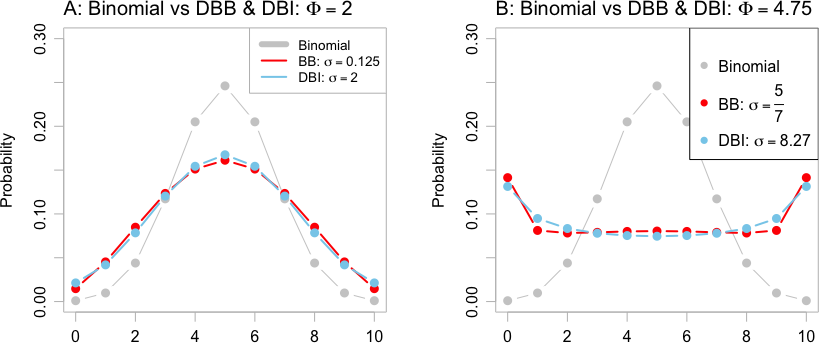Figure 2.1: Panel A compares the double binomial (DBI) and the betabinomial (BB) distribution with the dispersion parameter (DBI: $$\sigma = 2.0$$; BB: $$\sigma = 0.125$$) in each case chosen so that the dispersion index is $$\Phi = 2.0$$. Panel B repeats the comparison with the dispersion parameters (DBI: $$\sigma = 8.27$$; BB: $$\sigma = \frac{5}{7}$$) chosen so that $$\Phi = 4.75$$. The binomial distribution ($$\Phi$$ = 1), is shown for comparison, in both panels. As $$\Phi$$ increases, the change in shape needed to accomodate the increased variance becomes more extreme.

Note also the correlated binomial, implemented in the fitODBOD package, but with limited functionality for working with the fitted model. It is noted here in order to reinforce the point that there are multiple alternatives to the betabinomial.

### 2.1.1 The Pólya urn model as motivation for the betabinomial

The betabinomial is a generalization, allowing continuous parameter values, of the Pólya urn model. An urn holds $$\alpha$$ red balls and $$\beta$$ blue balls. In $$n$$ (= size or bd) draws, each ball that is withdrawn is replaced, prior to the next draw, by two balls of the same color. The effect is to move probability away from the mean or mode, and towards the extremes. See https://en.wikipedia.org/wiki/P%C3%B3lya_urn_model

### 2.1.2 Comparing the betabinomial with the quasibinomial

R’s glm() function offers the option of a quasibinomial error. Specification of a quasibinomial error has the consequence that the model is fitted as for a binomial distribution, with the the binomial variance $$n \pi (1- \pi)$$ then multiplied by a constant factor $$\Phi$$ that is usually estimated using the Pearson chi-squared statistic. For the betabinomial, the multiplier is $$\Phi = 1+(n-1)\rho$$, i.e., it increases with $$n$$. This is an important difference.

Figure 2.1 highlighted the extent to which the assumption of a distribution that has a binomial-like shape will be seriously wrong, if the dispersion index $$\Phi$$ is substantially greater than one. Whatever the distribution, if $$\Phi >> 1$$, the probability is pushed out towards the extremes, in ways that are sufficient to multiply the variance by the relevant factor $$\Phi$$.

## 2.2 Data that do, and do not, appear binomial

Figure 2.2 compares observed with fitted binomial counts, for two datasets. The data in Figure 2.2A appear close to binomial. That in Figure 2.2B shows clear differences from the binomial.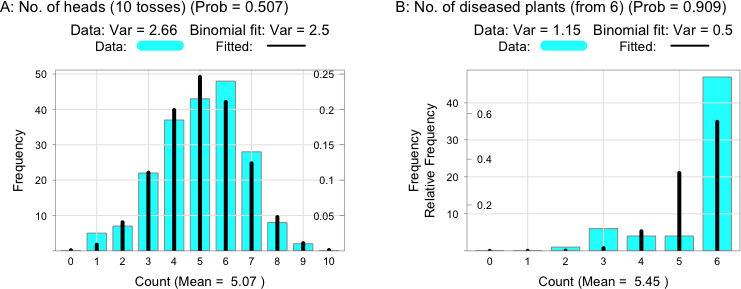Figure 2.2: In both panels, the vertical black lines show fitted values when a binomial distribution is fitted to the data. The data in Panel A has a distribution that is close to binomial. That in Panel B appears to deviate from binomial.

The Panel A data are the numbers of heads in 200 sets of ten coin tosses:

  A: Frequency of each of 0 to 10, in 10 tosses
0  1  2  3  4  5  6  7  8  9 10
0  5  7 22 37 43 48 28  8  2  0

The data can be obtained from the testDriveR package, thus

htab <- table(factor(
sapply(split(qra::kerrich,
rep(1:200,rep(10,200))),sum), levels=0:10),
dnn=list("A: Frequency of each of 0 to 10, in 10 tosses"))

The Panel B data are the numbers of diseased plants, out of 6, in 62 field quadrats.

  B: Frequency of each of 0 to 6, in 6 plants
0  1  2  3  4  5  6
0  0  1  6  4  4 47

The data can be obtained from the testDriveR package, thus

tastab <- table(factor(qra::rayBlight, levels=0:6),
dnn=list("B: Frequency of each of 0 to 6, in 6 plants"))

For data such as here, a poor fit to a binomial model is to be expected. The probability of disease is likely to vary from one quadrat to another, with some clustering of diseased plants within quadrats.

## 2.3 Diseased plants data — comparison of alternative models

The code that now follows was used to obtain binomial, double binomial, and betabinomial fits for the data, stored in the data frame diseased, that were used for Figure 2.2B. The fit is in each case handled as a special case of fitting a regression model. The only parameter is a location parameter — hence the ~1.

library(gamlss)
doBI <- gamlss(cbind(number, 6-number)~1, weights=freq,
family=BI, data=diseased, trace=FALSE)
doBB <- gamlss(cbind(number, 6-number)~1, weights=freq,
family=BB, data=diseased, trace=FALSE)
doDBI <- gamlss(cbind(number, 6-number)~1, weights=freq,
family=DBI, data=diseased, n.cyc=100, trace=FALSE)

Now examine, and compare, diagnostic plots for the binomial (Figure 2.3), and for the betabinomial (Figure 2.4) model. Each plot is based on six sets of randomized quantile residuals (howmany=6 is the default), while the setting plot.type="all" for Panels A and B has the effect that all points are shown (in gray), while the medians are shown as solid black dots. Panel B (a ‘worm plot’) is a detrended version of Panel A, with the dashed curves marking out 95% confidence bounds.

Figure 2.3 shows diagnostic plots for a binomial distribution: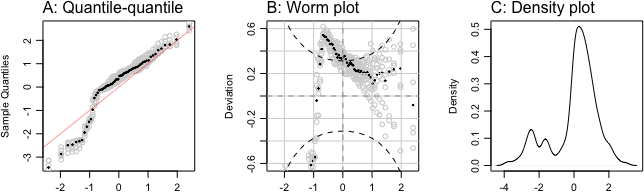Figure 2.3: Diagnostic plots of randomized quantile residuals (identified as sample quantiles), for a binomial model fitted to the plant disease data. Panel B (a ‘worm plot’) is a detrended version of Panel A, with the dotted curves marking out 95% confidence bounds.

Figure 2.4 shows diagnostic plots for a betabinomial distribution: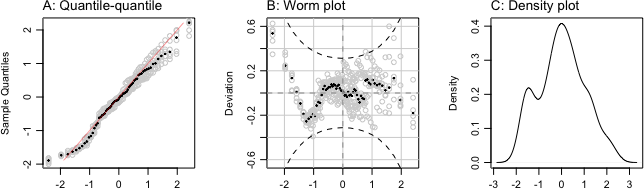Figure 2.4: Diagnostic plots of randomized quantile residuals, for a betabinomial model fitted to the plant disease data.

2.4 The worm plots give the clearest picture. Figure 2.3B makes it clear that the data is not binomial, while Figure 2.4B indicates that the data are broadly consistent with a betabinomial distribution.

Code is:

## Binomial fits
rqres.plot(doBI, plot.type='all', type="QQ")
res <- rqres.plot(doBI, plot.type='all', type="wp")
plot(density(res), xlab="Quantile residuals", main="")

Replace doBI by doBB, for the code for the betabinomial diagnostic plots.

The AIC statistic can be used for a theoretically based comparison between model fits. As with other such ‘information’ statistics, the AIC statistic is not designed to provide statistical tests. The following uses the AIC statistic to compare the three models that have been fitted – the binomial, the betabinomial, and the double binomial (for which diagnostic plots have not been shown.) The values given in the dAIC column are increases from the best fitting model.

aicStat <- AIC(doBI,doBB,doDBI)
newnames <- c(doBI="Binomial", doBB="Betabinomial", doDBI="Double binomial")
rownames(aicStat) <- as.vector(newnames[rownames(aicStat)])
aicStat[,'dAIC'] <- c(0,diff(aicStat[,'AIC']))
print(aicStat)
df   AIC   dAIC
Betabinomial     2 118.9  0.000
Double binomial  2 122.6  3.703
Binomial         1 152.1 29.560

The comparison favors the betabinomial, by a smallish margin.

### Do differences from the binomial matter?

Do the differences matter? That depends on the use that will be made of the results. If the use of the model depends on accurately predicting the proportion of diseased samples, the differences clearly will matter. A confidence interval, e.g., for a difference between two different sample areas, may be acceptably accurate provided that normal approximations are used for the sampling distributions of the two means, and the variances are calculated from the data. For this, we are relying on Central Limit Theorem effects (see ??) to bring the sampling distribution of the mean close to the normal.

## 2.4 Numbers of males, in first 12 of 13 children

Data from large families indicates that variation in the proportion of males is greater than would be expected for a binomial distribution. The dataset qra::malesINfirst12, from hospital records in Saxony in the nineteenth century, gives the number of males among the first 12 children of family size 13 in 6115 families. The probability that a child will be male varies, within and/or between families. (The 13th child is ignored to counter the effect of families non-randomly stopping when a desired gender is reached.) Data is:

maleDF <- qra::malesINfirst12
## Numbers of male children, out of 12
as.table(setNames(maleDF$freq, nm=0:12)) 0 1 2 3 4 5 6 7 8 9 10 11 12 3 24 104 286 670 1033 1343 1112 829 478 181 45 7  The following fits the model with binomial errors. if(PKGgamlss){ doBI <- gamlss(cbind(No_of_Males, 12-No_of_Males)~1, weights=freq, family=BI, data=maleDF, trace=FALSE) doBB <- gamlss(cbind(No_of_Males, 12-No_of_Males)~1, weights=freq, family=BB, data=maleDF, trace=FALSE) doDBI <- gamlss(cbind(No_of_Males, 12-No_of_Males)~1, weights=freq, family=DBI, data=maleDF, trace=FALSE, n.cyc=100) } For the betabinomial (doBB), replace family=BI by family=BB. For the double binomial (doDBI), replace family=BI by family=DBI. Fitted probabilities for the betabinomial can, if required, be calculated and added to the data frame maleFit thus: if(PKGgamlss){ maleDF$BBfit <- with(maleDF, dBB(No_of_Males, bd=12,
mu = fv(doBB, 'mu'),
sigma=fv(doBB, 'sigma')))
maleDF$DBIfit <- with(maleDF, dBB(No_of_Males, bd=12, mu = fv(doDBI, 'mu'), sigma=fv(doDBI, 'sigma'))) } else { maleDF$BBfit <- c(0.000384,0.003692,0.017142,0.050837,0.10723,0.169453,
0.205716,0.193319,0.139586,0.075539,0.02909,0.00716,0.000852)
maleDF\$DBIfit <- c(0.168803,0.079223,0.060521,0.052428,0.048321,0.046371,
0.045942,0.046893,0.049449,0.054383,0.063858,0.085812,0.197996)
}

The code can be modified in the obvious way to add fitted values for the binomial and/or the double binomial.

Figure 2.5 shows worm plots for the binomial and betabinomial models: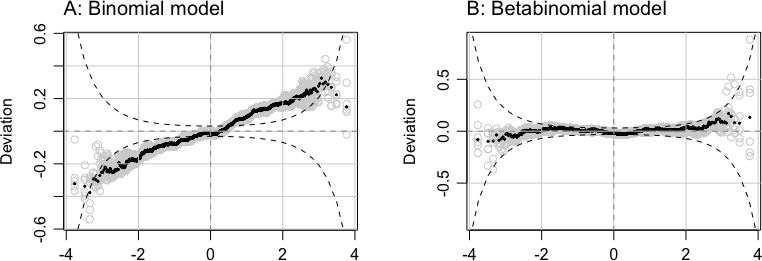Figure 2.5: Data, from hospital records in Saxony in the nineteenth century, gave the number of males among the first 12 children in families of size 13, in 6115 families. Panel A shows a worm plot for the model that fitted a binomial distribution. Panel B repeats the worm plot, now for the model that fitted a betabinomial distribution.

The Panel A plot, with points all lying close to a line, indicates that data follow a binomial-like pattern of variation. The upward slope of the line indicates that points are more dispersed than for a binomial distribution. The Panel B plot indicates that data are consistent with the betabinomial fit from which this plot was generated.

Code for the plots is:

oldpar <- par(mar=c(2.6,4.1,2.6,1.6), mgp=c(2.5,.75,0), mfrow=c(1,2))
mtext(side=3, line=0.75, "A: Binomial model", adj=0, cex=1.25)

AIC statistics for the three models are:

                df   AIC dAIC
Double binomial  2 24988  0.0
Betabinomial     2 24990  1.3
Binomial         1 25070 81.9

The double binomial is by a small and inconsequential margin the preferred model.

### Bernoulli 0/1 data are a special case

Note that for data with a 0/1 response, neither the quasibinomial nor the betabinomial or other such model can be fitted, unless the 0/1 responses can be grouped to give repeated $$x$$ out of $$n$$ sets of results. With a 0/1 response, the residual deviance is a function of the fitted parameters, and gives no information on the variance. See McCullagh and Nelder (1989), Section 4.5.1.